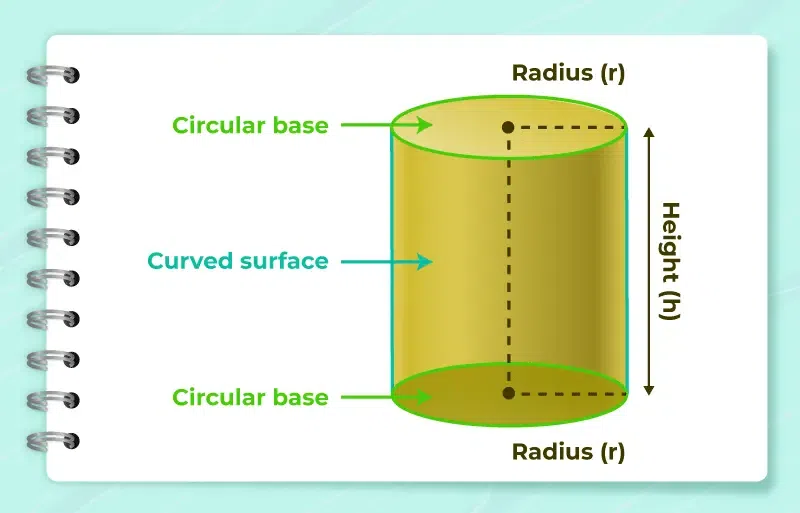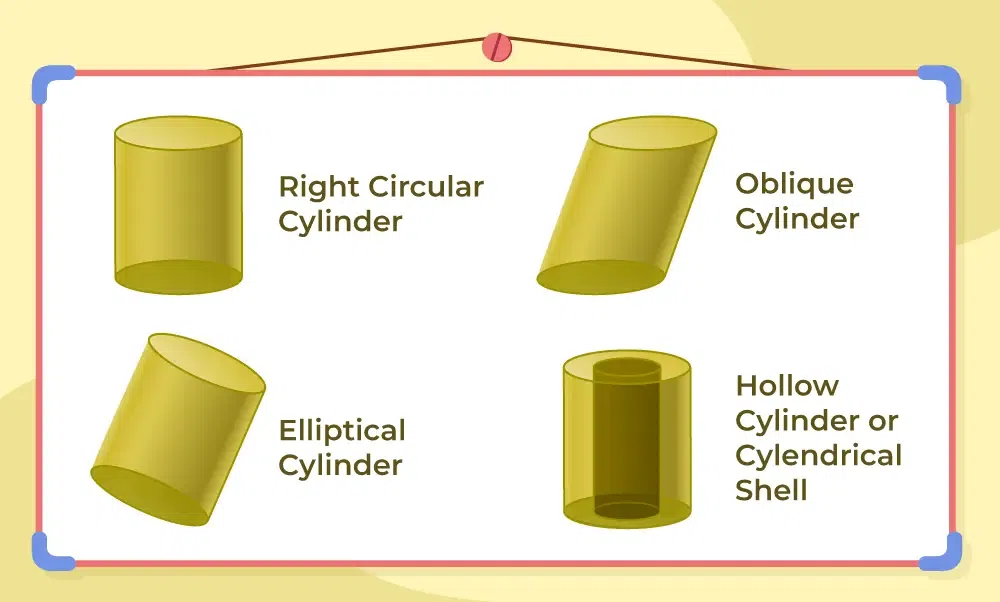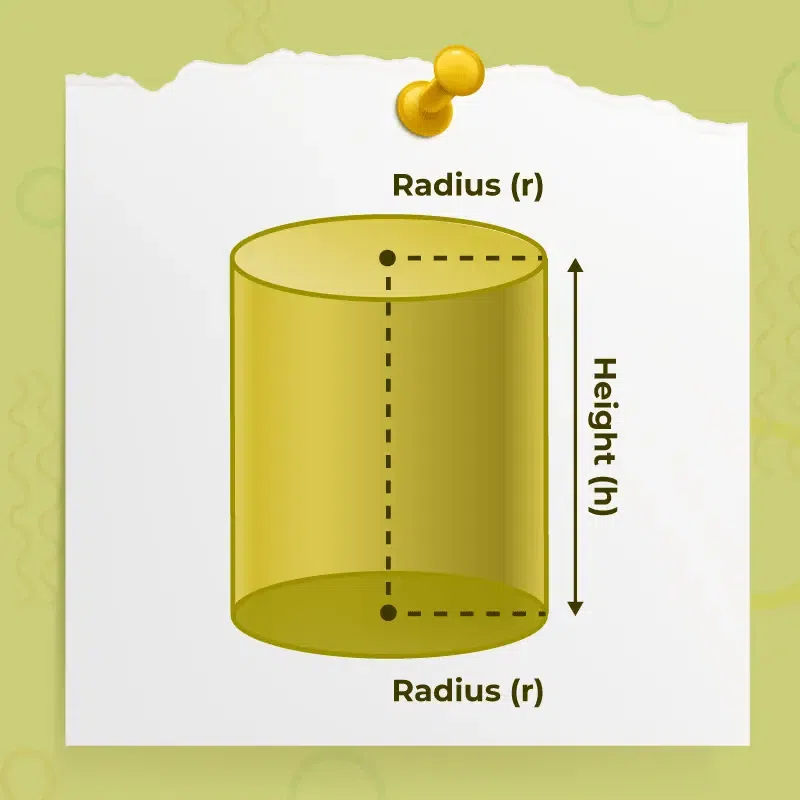GFG App
Open AppBrowser
Continue

Cylinder is a three-dimensional shape with two parallel circular discs or bases separated by a certain distance. The word “cylinder” originates from the Greek word “kylindros” which means “roll” or “roller. Various examples which we regularly observed are toilet paper rolls, cold drink cans, gas cylinders, and many more.

The cylinder has height denoted by h which is defined as the distance between the two parallel circular bases of the cylinder. The radius of the cylinder is defined as the radius of its base. So, let’s learn about the cylinder in detail including topics such as its shape, surface area, and volume.

## Cylinder Definition

A cylinder is a three-dimensional shape with two circular bases connected by a lateral surface known as the curved surface. The distance between the two parallel circular bases of the cylinder is referred to as the height (h) of the cylinder and the line connecting the centres of the two circular bases is the axis of the cylinder. The radius (r) of a cylinder is the distance from the centre to the outer boundary of a cylinder.## Cylinder Shape

A cylinder is a three-dimensional shape consisting of two parallel circular bases, joined by a curved surface. The centre of the circular bases overlaps each other to form a right cylinder. The line segment joining the two centres is the axis, which denotes the height of the cylinder.

The top view of the cylinder looks like a circle and the side view of the cylinder looks like a rectangle. Unlike cones, cubes, and cuboids, a cylinder does not have any vertices, since the cylinder has a curved shape and no straight lines. It has two circular faces.

## Cylinder Faces Vertices Edges

Any 3-D figure has faces, vertices, and edges which define the features of that figure. The two circular faces of the cylinder are parallel to each other and the distance between them is the height of the cylinder. Faces, vertices and edges in the cylinder are:

• Faces: In a cylinder, there are a total of 3 faces i.e. 2 flat circular faces + 1 curved face
• Edges: In a cylinder, there are 2 circular edges one at the top face and one at the bottom face.
• Vertices: In a cylinder, there are 0 vertices.

## Properties of Cylinder

Cylinder has some properties that differentiate it from other shapes. Some of those properties of the cylinder are:

• Bases of cylinders are always congruent and parallel to each other.
• A cylinder has two flat faces which are identical to each other and one curved surface.
• Volume and Area of any cylinder are proportional to the radius and height of the cylinder.

## Types Of Cylinder

Cylinders are classified into various types, such as right circular cylinders, Oblique cylinders, Elliptical cylinders, and cylindrical shells or hollow cylinders.• Right Circular Cylinder: A right circular cylinder is a cylinder with an axis of the cylinder perpendicular to the centre of the base.
• Oblique cylinders: An oblique cylinder is a cylinder with the axis of the cylinder not perpendicular to the centre of the base, i.e., the axis makes an angle other than a right angle with the centre of the base.
• Elliptical cylinders: An elliptical cylinder is a cylinder with elliptical-shaped bases.
• Cylindrical shells or hollow cylinders: A hollow cylinder is made up of two right-circular cylinders that are bound inside one another. It is void from the inside and has a difference between the inner and outer radii. The point of the axis is perpendicular to the central base and common to both cylinders. Some real-life examples of hollow cylinders are hollow pipes, toilet paper rolls, etc.

## Cylinder Formulas

Like every other three-dimensional geometric shape, even a cylinder has two major formulae, i.e., surface area and volume. A cylinder has two kinds of surface areas: the curved surface area or the lateral surface area, and the total surface area.

So, the three major formulae related to a cylinder are,

• Curved Surface Area of Cylinder
• Total Surface Area of Cylinder
• Volume of Cylinder## Curved Surface Area of Cylinder

The curved surface area, or lateral surface area of a cylinder, is the space enclosed between the two parallel circular bases.

The formula for the curved surface area, or lateral surface area, of a cylinder, is given as,

Curved Surface Area (CSA) = Circumference × Height

CSA = 2πr × h = 2πrh square units

Curved Surface Area (CSA) = 2πrh

Where,

• r is the radius of the cylinder, and
• h is the height of the cylinder.

## Total Surface Area of Cylinder

The total surface area of a cylinder is the total area enclosed by a cylinder, including its bases. A cylinder has a lateral surface and two bases that are parallel and identical. Therefore, the total surface area of a cylinder is the sum of the area of the curved surface or lateral surface and the areas of the two circular bases.

We know that,

Curved Surface Area (CSA) = (2 π r h) square units

Area of a Circle = πr2 square units

Total Surface Area (TSA) of a cylinder = Curved Surface Area + 2(Area of a circle)

TSA = 2πrh + 2πr2 = 2πr(h + r) square units

Thus, the formula for the total surface area of a cylinder is given as,

Total Surface Area (TSA) = [2πr(h + r)] square units

Where,

• r is the radius of the cylinder, and
• h is the height of the cylinder.

## Volume of Cylinder

The volume of a cylinder is the density or amount of space occupied by the cylinder. Let us assume that a cylindrical-shaped container is filled with refined oil. Now, to calculate the amount of oil, we need to determine the volume of the cylindrical-shaped container.

Now, the volume of a cylinder = Area of a circle × height

Volume (V) = πr2 × h cubic units

Thus, the formula for the volume of a cylinder is given as,

Volume of a cylinder =  (πr2h) cubic units

Where,

• r is the radius of the cylinder, and
• h is the height of the cylinder.

## Net of a Cylinder

The net of any 3-D figure is the 2-D structure which is made by cutting or opening the 3-D figure. It is used to visualise the area of the 3-D figure and helps in the construction of the 3-D figure.

For a cylinder, the net is a 2D structure made by opening it. Opening or unfolding a cylinder results in a rectangle joined by two identical circles which act as the top and bottom of the cylinder.

Also, Check

## Solved Examples on Cylinder

Example 1: Determine the curved surface area of the cylinder with a radius of 8 inches and a height of 15 inches.

Solution:

Given,

The height of the cylinder = 15 inches.

We have,

The curved surface area of the cylinder = (2πrh) square units

⇒ CSA of Cylinder = 2 × (22/7) × 8 × 15

⇒ CSA of Cylinder = 754.285 sq. in

Hence, the curved surface area of the cylinder is 754.285 sq. in.

Example 2: Calculate the volume of a cylindrical-shaped water container that has a height of 18 cm and a diameter of 12 cm.

Solution:

Given: Height = 18 cm,

Diameter = 12 cm

As Diameter = 2 × radius,

⇒ 2 × radius = 12 cm

⇒ r = 6 cm

We have,

Volume of Cylinder =  (π r2 h) cubic units

⇒ V = (22/7) × (6)2 × 18

⇒ V = 2,034.72‬ cm3

Hence, volume of the cylindrical-shaped water container is 2034.72 cm3.

Example 3: Determine the total surface area of the cylinder with a radius of 6 cm and a height of 12 inches.

Solution:

Given: Radius = 6 cm and Height = 12 cm

We have,

Total surface area of the cylinder = [2πr(h + r)]

⇒ Total surface area of the cylinder = 2 × (22/7) × (6) × (6 + 12)

⇒ Total surface area of the cylinder = 37.714 × 18

⇒ Total surface area of the cylinder = 678.857 sq. cm.

Therefore, total surface area of the cylinder is 678.857 sq. cm.

Example 4: Determine the height of the cylinder if its volume is 625 cubic units and its radius is 5 units.

Solution:

Given: Volume = 625 cubic units, and

Let the height of the cylinder be h units.

We know that, Volume of a cylinder =  π r2 h

⇒ 625 = (22/7) × (5)2 × h

⇒ h = (625/25) × (7/22)

⇒ h = 7.95 units

Hence, height of the given cylinder is 7.95 units

Example 5: Determine the curved surface area of a cylinder if its total surface area is 770 sq. in and its radius is 7 inches.

Solution:

Given: Radius = 7 inches, and

Total Surface Area of the cylinder = 770 sq. in

We know that,

Total surface area of a cylinder = [2πr(h + r)] square units

⇒ 770 = 2 × (22/7) × (7) × (7 + h)

⇒ 2 × (22) × (7 + h) = 770

⇒ 7 + h = 17.5‬

⇒ h = 10 inches

Now,

⇒ Curved surface area of cylinder = 2πrh square units

⇒ Curved surface area of cylinder = 2 × (22/7) × (7) × (10)

⇒ Curved surface area of cylinder = 2 × (22) × (10)

⇒ Curved surface area of cylinder = 440 sq. in.

Thus, curved surface area of the given cylinder is 440 sq. in.

Example 6: Find the radius of the cylinder if its curved surface area is 550 sq. cm and its height is 14 cm.

Solution:

Given: Curved Surface Area of cylinder = 550 sq. cm, and

Height of Cylinder = 14 cm

Let the radius of cylinder be r cm.

We know that, Curved Surface Area of the cylinder = 2πrh

⇒ 550 = 2 × (22/7) × r × 14

⇒ 88r = 550

⇒ r = 550/88

⇒  r = 6.25 cm

Hence, radius of ‬the given cylinder is 6.25 cm.

## FAQs on Cylinder

### Q1: What is a Cylinder?

Cylinder is one of the basic 3-D figure which we see very often in our daily life such as cold drink cans, cylinders, rollers, etc. It comprises two circular surfaces and a curved surface.

### Q2: What are the Cylinder Formulas?

A cylinder has various formulas, some of the important formulas of a cylinder are

• Total Surface Area = 2πr(r+h) units2
• Curved Surface Area = 2πrh units2
• Volume = πr2h units3

### Q3: What is the volume of a cylinder?

Volume of Cylinder is defined as the total capacity any cylinder can hold.

### Q4: How many vertices and edges does a cylinder have?

A cylinder has zero vertices and two edges.

### Q5: What are real-life examples of cylinders?

Some Real-life examples of cylinders are Gas cylinders, Cans, Pipes, Water Bottel, etc.

### Q6: What is the formula for the volume of a cylinder?

The formula for finding the volume of a cylinder is:

Volume of Cylinder = πr2h cubic units